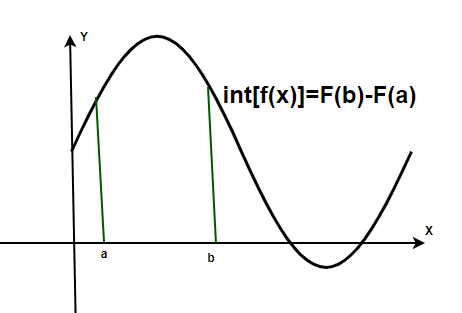Open in App
Not now

# Evaluating Definite Integrals

• Last Updated : 20 May, 2021

Integration, as the name suggests is used to integrate something. In mathematics, integration is the method used to integrate functions. The other word for integration can be summation as it is used, to sum up, the entire function or in a graphical way, used to find the area under the curve function. Integration is known to be the opposite of differentiation which is the process of breaking down a function into smaller functions whereas integration is summing the smaller bits to obtain the entire area under the curve.

### Definite Integrals

There are two ways of integration possible- definite integration and indefinite integration. Definite integration is done for the area given with limits or boundaries, that is, the curve is finite and hence, the area under the curve shall also be finite whereas indefinite integration is used for the function having no upper or lower limits, the functions are infinite in nature and therefore, the upper and lower limits for indefinite functions are +∞ and -∞.

Definite integrals of a function f(x) from a to b when the function f is continuous in the closed interval [a, b].Where, a and b are the lower and upper limits.

F(x) is the integral of f(x), and if f(x) is differentiated, F(x) is obtained. Hence, it can be said F is the anti-derivative of f. Definite integrals are also known as Riemann Integrals.### Evaluation of Definite Integrals — Properties

In Mathematics, simple summation and addition are easy but when it comes to evaluating complex integrals, simple calculations and additions are not enough, therefore, Integration is required. While evaluating definite integrals, sometimes calculations become too cumbersome and complex, so some empirically proven properties are made in order to make the calculations comparatively easy.

Property 1:This property can be proved by simply substituting the value of “t” in place of “x”.

Property 2:Proof: Let F(x) be anti-derivative of the integralSo, we knowComparing these two equations, we can deduce thatHence, Proved.

Property 3:Proof:Adding the above two equations will give us the first equation,Property 4:Proof: Let’s assume t = a + b -x. Then dt = -dx, When x = a, t = b and wh

en x = b, t = a.

Therefore,Property 5:Proof: This property is a particular case property 4, so it can also be proved that way.

Property 6:Proof: Using Property 3, we can write this as,Let t = 2a  x in the second integral on the right-hand side. Then dt = – dx. When x = a, t = a and when x = 2a, t = 0. Also, x = 2a – t. Therefore, the second integral becomesHence, \Property 7:Proof: Using property 6, we haveNow, if f(2a – x) = f(x) then this equation becomes,And if f(2a – x) = – f (x), then that equation becomesProperty 8:if it’s an even function, i.e, if f(-x) = f(x).

Property 9:, if it’s an odd function, i.e f(-x) = -f(x).

Proof: Using Property 3, we have,Let t = – x in the first integral on the right-hand side.

dt = – dx. When x = – a, t = a and when x = 0, t = 0. Also, x = – t.

So, we can rewrite the above expression as,1. Now, if f is an even function i.e f(-x) = f(x),2. If f is an odd function i.e f (–x) = – f(x).### Sample Problems

Question 1: Evaluate,Solution:

Integrating,Question 2: Evaluate,Solution:

Integrating the above given function,Question 3: Evaluate,Solution:

Integrating the function,Question 4: Given,. Find the value of.

Solution:

For solving this question, we will be using Property 3.Now, we just have to plug in the value of the given definite integrals.Question 5: Given f(x) = x3. Find the value of.

Solution:

Now this answer can be calculated through usual integration method, it is fairly easy. But we can think of a property which can be used here to completely save your calculation and time.

Property 8 and Property 9 can be used depending upon which kind of function f(x) = x3 is, even or odd. Let’s check whether f(x) is even or odd.

For an even function, f(-x) = f(x) and for an odd function f(-x) = -f(x).

Here in our case, f(-x) = (-x)3 = -x3 = -f(x). So it is an odd function.

Thus, Property 9 is applicable here, and through property 9 we can say that.Question 6: Given,. Find the value ofSolution:

This question is similar to what we solved a little while ago, It also requires application of Property 3. But we need to do something aboutThis problem can be solved by the application of Property 2.

Using property 2,So, now plugging the values,Question 7: Evaluate,Solution:

In previous questions we checked for an even or odd function. By a similar check, we can say that sin2x is an even function.

So by the use property 8, we can rewrite it as,Question 8: Evaluate,Solution:

This expression contains two terms. We know,

Sin(x) is an odd function and x4 is an even function.

So,My Personal Notes arrow_drop_up
Related Articles